Theta graph

In computational geometry, the Theta graph, or -graph, is a type of geometric spanner similar to a Yao graph. The basic method of construction… Expand
Wikipedia

Papers overview

Semantic Scholar uses AI to extract papers important to this topic.
2019
2019
• Graphs and Combinatorics
• 2019
With respect to specific cycle-related problems, edge-colored graphs can be considered as a generalization of directed graphs. We… Expand
•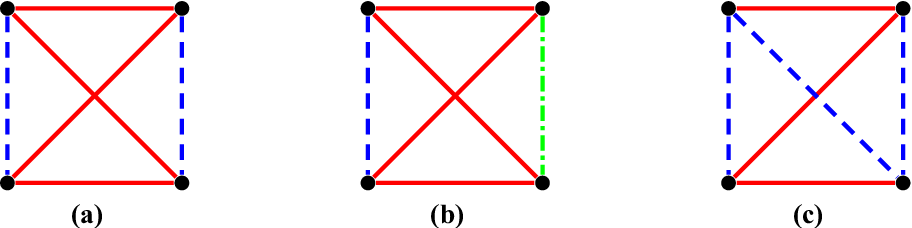•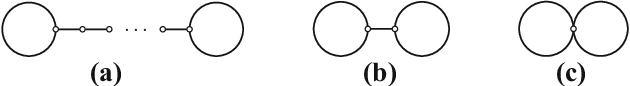•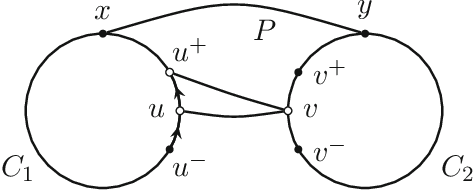•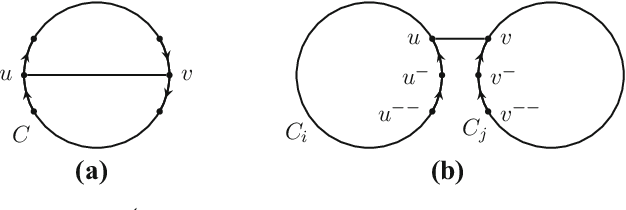•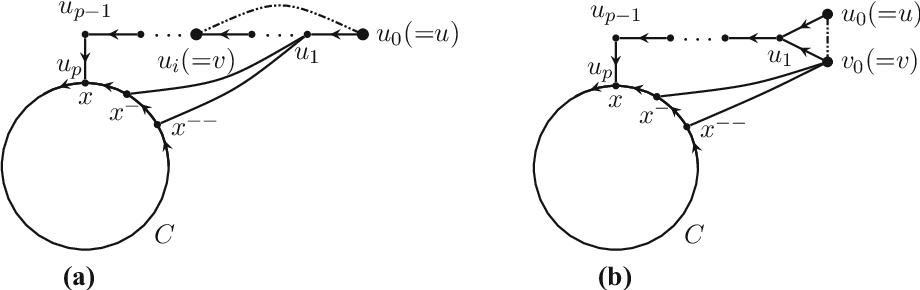Is this relevant?
2018
2018
• 2018
The theta graph $\Theta_{\ell,t}$ consists of two vertices joined by $t$ vertex-disjoint paths of length $\ell$ each. For fixed… Expand
Is this relevant?
2018
2018
AbstractThe Kinoshita graph is a particular embedding in the 3-sphere of a graph with three edges, two vertices, and no loops. It… Expand
•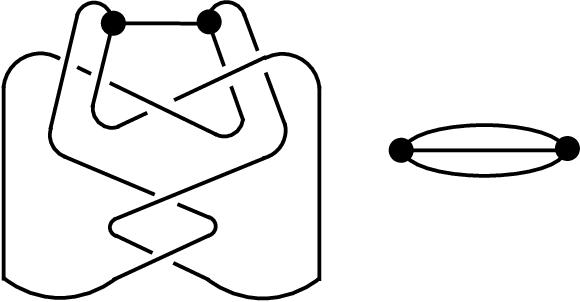•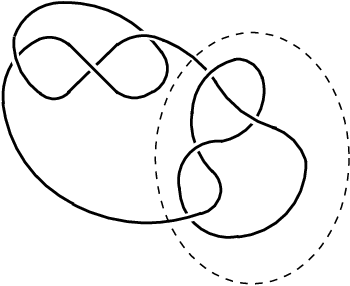•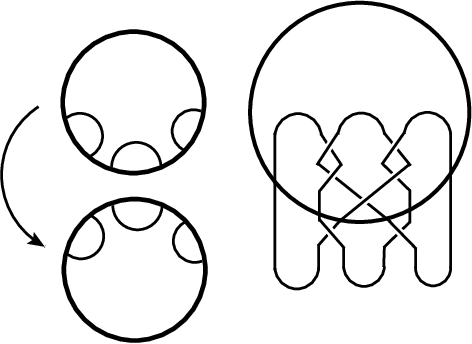•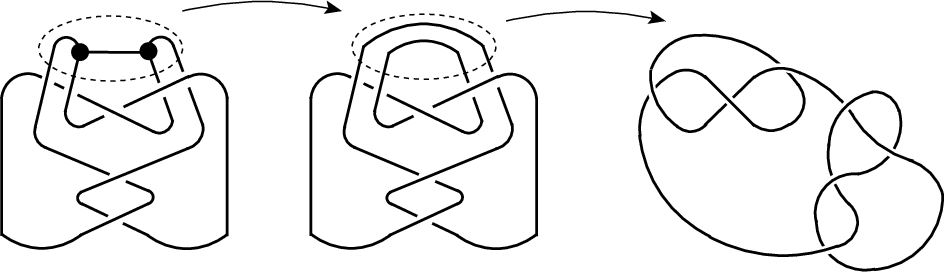•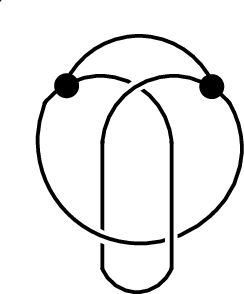Is this relevant?
2017
2017
• 2017
Abstract. A sum divisor cordial labeling of a graph G with vertex set V is a bijection f from V to {1, 2,..., | |} V such that… Expand
•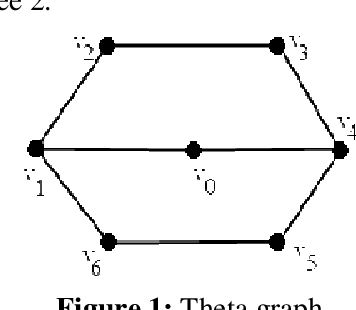•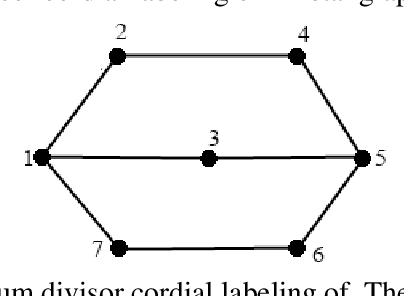•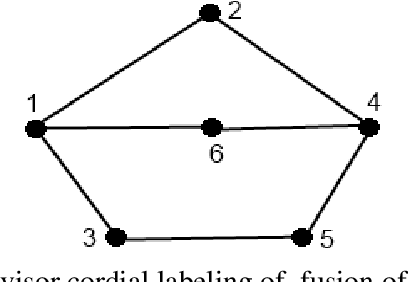•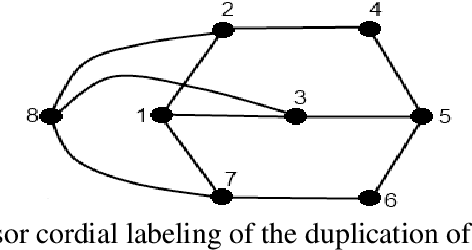•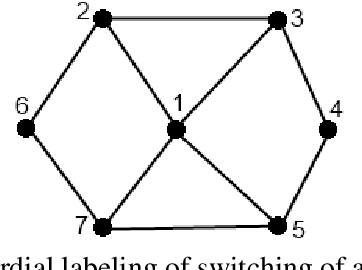Is this relevant?
2015
2015
• Graphs and Combinatorics
• 2015
In online list coloring [introduced by Zhu (Electron J Comb 16(1):#R127, 2009) and Schauz (Electron J Comb 16:#R77, 2009)], on… Expand
Is this relevant?
2012
2012
• Discrete Math., Alg. and Appl.
• 2012
Yao and Theta graphs are defined for a given point set and a fixed integer k > 0. The space around each point is divided into k… Expand
•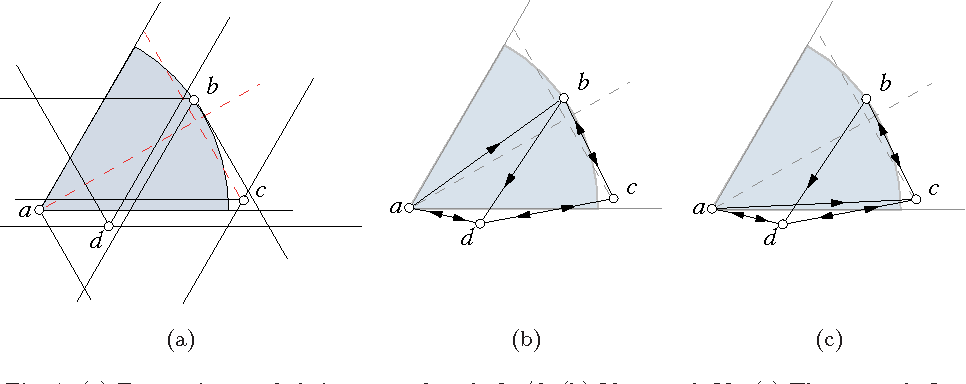•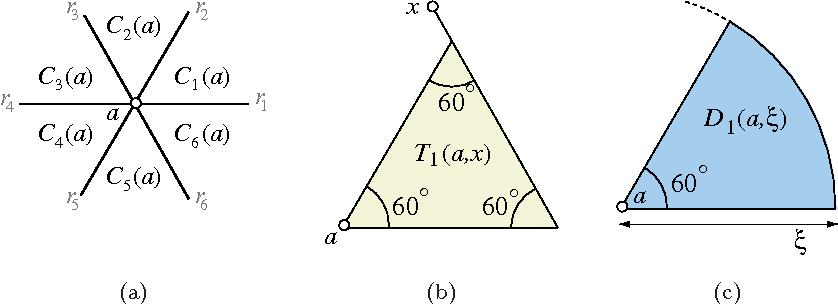•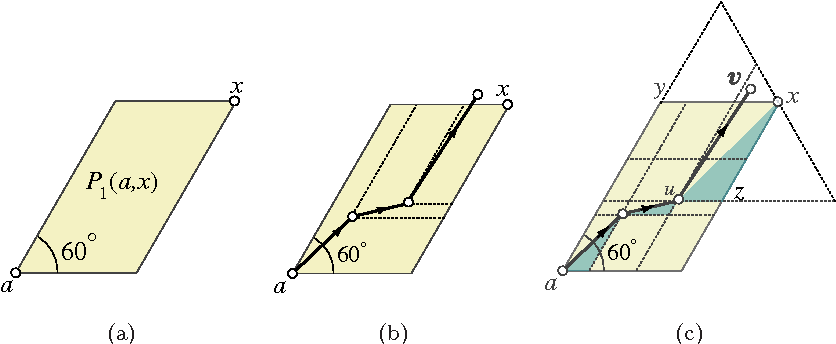•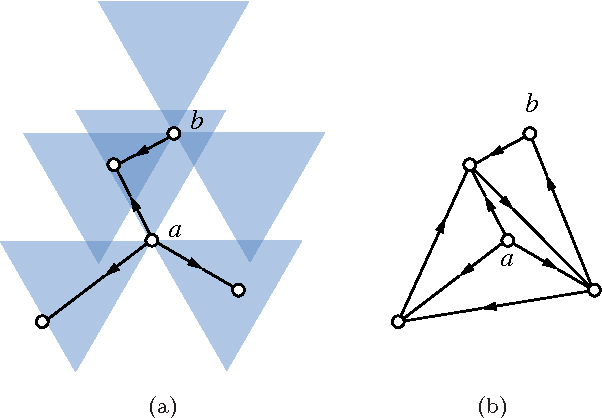•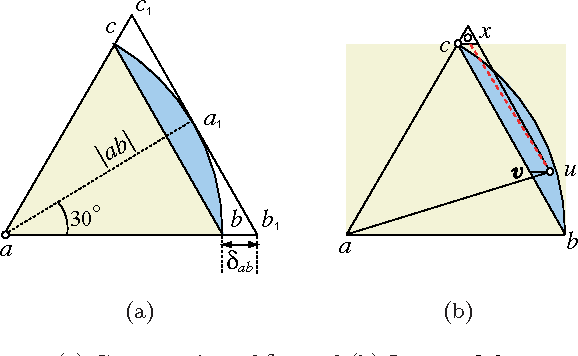Is this relevant?
2010
2010
• COCOA
• 2010
The Yao and Theta graphs are defined for a given point set and a fixed integer k > 0. The space around each point is divided into… Expand
Is this relevant?
2007
2007
• Discrete Applied Mathematics
• 2007
Given a graph G, an edge-coloring of G with colors 1,2,3,... is consecutive if the colors of edges incident to each vertex are… Expand
Is this relevant?
2001
2001
We discuss various constructions which allow one to embed a principally polarized abelian variety in the jacobian of a curve… Expand
Is this relevant?
2000
2000
• J. Comb. Theory, Ser. B
• 2000
The generalized theta graph \Theta_{s_1,...,s_k} consists of a pair of endvertices joined by k internally disjoint paths of… Expand
Is this relevant?